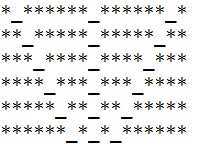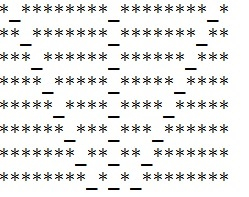# C++ Program for Expressionless Face Pattern printing

C++Server Side ProgrammingProgramming

#### C in Depth: The Complete C Programming Guide for Beginners

45 Lectures 4.5 hours

#### Practical C++: Learn C++ Basics Step by Step

Most Popular

50 Lectures 4.5 hours

#### Master C and Embedded C Programming- Learn as you go

66 Lectures 5.5 hours

Given a number n; the task is to create the Expressionless face pattern upto n lines and display the results. Expressionless face is created using special characters, expressionless face using special character seems like: “*_*”.

## Example

Input-: n = 6
Output-:Input-: n = 8
Output-:## Algorithm

Start
Step 1-> In function print_stars(int i)
Loop For j = 1 and j <= i and j++
Print “*”
Step 2-> In function print_pattern(int rows)
Loop For i = 1 and i <= rows and i++
Call function print_stars(i)
Print “_”
Call print_stars(rows - i + 1)
Print “_”
Call print_stars(rows - i + 1)
Print ”_”
Call print_stars(i)
Print newline
Step 3-> In function int main()
Declare and set rows = 8
Call print_pattern(rows)
Stop

## Example

Live Demo

#include <bits/stdc++.h>
using namespace std;
//function to print stars
void print_stars(int i) {
for (int j = 1; j <= i; j++)
cout << "*";
}
void print_pattern(int rows) {
for (int i = 1; i <= rows; i++) {
print_stars(i);
cout << "_";
print_stars(rows - i + 1);
cout << "_";
print_stars(rows - i + 1);
cout << "_";
print_stars(i);
cout << endl;
}
}
int main() {
int rows = 8;
print_pattern(rows);
return 0;
}

## Output